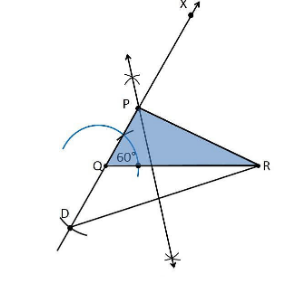# Construct a triangle PQR in which QR = 6cm, ∠Q = 60° and PR-PQ = 2cm.

PProcedure

1. Draw a line segment of base QR = 6 cm

2. Measure and draw ∠Q = 60° and let the ray be QX

3. Using a compass measure PR–PQ = 2cm.

4. As PR–PQ is negative, QD will below the line QR.

5. With Q as a centre and draw an arc at the point be D on the ray QX

6. Join DR

7. Draw the perpendicular bisector of the line DR and the intersection point is taken as P.

8. Join PR

9. Hence, PQR is the required triangle.